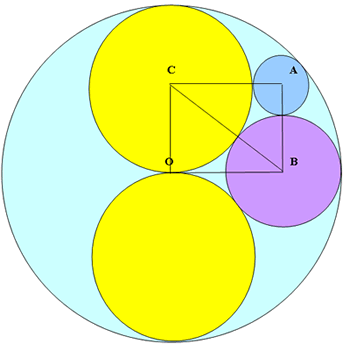#### You may also like### Baby Circle

A small circle fits between two touching circles so that all three circles touch each other and have a common tangent? What is the exact radius of the smallest circle?### Kissing

Two perpendicular lines are tangential to two identical circles that touch. What is the largest circle that can be placed in between the two lines and the two circles and how would you construct it?### Logosquares

Ten squares form regular rings either with adjacent or opposite vertices touching. Calculate the inner and outer radii of the rings that surround the squares.

# Retracircles

##### Age 16 to 18Challenge Level

The circles centres $O$, $A$, $B$ and $C$ touch one another. Prove that the radii of the circles with centres $A$, $B$ and $C$ are in the ratio $1 : 2 : 3$ and that the triangles $OBC$ and $ABC$ are right angled $3 : 4 : 5$ triangles.